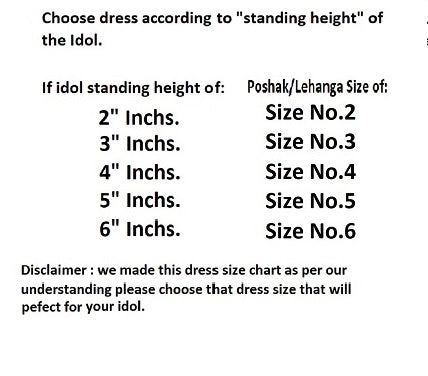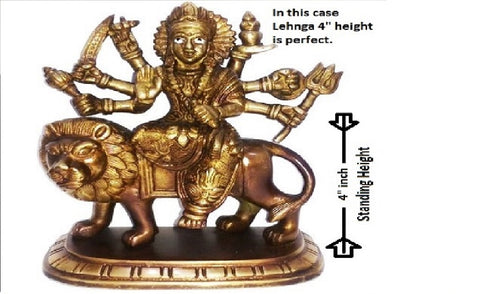We are accepting prepaid orders only. Delivery time: Delhi/NCR( 1-3 days) and Other Locations (6 to 7 days).

# Collection: Mata Ki Dresses205 products
• Mata Rani _Poshak-Lehenga Size - (2" Inch.)
Regular price
Rs. 85.00
Sale price
Rs. 85.00
Unit price
per
• Mata Rani _Poshak-Lehenga Size - (2" Inch.)
Regular price
Sold out
Sale price
Rs. 70.00
Unit price
per
• Mata Rani _Poshak-Lehenga Size - (3" Inch.)
Regular price
Sold out
Sale price
Rs. 65.00
Unit price
per
• Mata Rani _Poshak-Lehenga Size - (3" Inch.)
Regular price
Sold out
Sale price
Rs. 85.00
Unit price
per
• Mata Rani _Poshak-Lehenga Size - (4" Inch.)
Regular price
Rs. 150.00
Sale price
Rs. 150.00
Unit price
per
• Mata Rani _Poshak-Lehenga Size - (6" Inch.)
Regular price
Rs. 300.00
Sale price
Rs. 210.00
Unit price
per
• Mata Rani _Poshak-Lehenga Size - (3" Inch.)
Regular price
Rs. 75.00
Sale price
Rs. 75.00
Unit price
per
• Mata Rani _Poshak-Lehenga Size - (2" Inch.)
Regular price
Rs. 75.00
Sale price
Rs. 75.00
Unit price
per
• Mata Rani _Poshak-Lehenga Size - (5" Inch.)
Regular price
Rs. 175.00
Sale price
Rs. 175.00
Unit price
per
• Mata Rani _Poshak-Lehenga Size - (6" Inch.)
Regular price
Rs. 250.00
Sale price
Rs. 199.00
Unit price
per
• Mata Rani _Poshak-Lehenga Size - (3" Inch.)
Regular price
Rs. 85.00
Sale price
Rs. 85.00
Unit price
per
• Mata Rani _Poshak-Lehenga Length - 4" Inch's
Regular price
Rs. 125.00
Sale price
Rs. 125.00
Unit price
per
• Mata Rani _Poshak-Lehenga Size - (4" Inch.)
Regular price
Rs. 120.00
Sale price
Rs. 120.00
Unit price
per
• Mata Rani _Poshak-Lehenga Size - (4" Inch.)
Regular price
Rs. 75.00
Sale price
Rs. 75.00
Unit price
per
• Mata Rani _Poshak-Lehenga Size - (3" Inch.)
Regular price
Rs. 140.00
Sale price
Rs. 140.00
Unit price
per
• Mata Rani _Poshak-Lehenga Size - (2" Inch.)
Regular price
Rs. 140.00
Sale price
Rs. 100.00
Unit price
per
• Mata Rani _Poshak-Lehenga Size - (3" Inch.)
Regular price
Rs. 160.00
Sale price
Rs. 120.00
Unit price
per
• Mata Rani _Zari Poshak- Lehenga Length - (5" Inch.)
Regular price
Sold out
Sale price
Rs. 550.00
Unit price
per
• Mata Rani _Poshak-Lehenga Size - (5" Inch.)
Regular price
Sold out
Sale price
Rs. 220.00
Unit price
per
• Mata Rani _Poshak-Lehenga Size - (3" Inch.)
Regular price
Sold out
Sale price
Rs. 160.00
Unit price
per
• Mata Rani _Poshak-Lehenga Size - (5" Inch.)
Regular price
Rs. 199.00
Sale price
Rs. 199.00
Unit price
per
• Mata Rani _Poshak-Lehenga Size - (4" Inch.)
Regular price
Rs. 175.00
Sale price
Rs. 175.00
Unit price
per
• Mata Rani _Poshak-Lehenga Size - (3" Inch.)
Regular price
Rs. 145.00
Sale price
Rs. 145.00
Unit price
per
• Mata Rani _Poshak-Lehenga Size - (5" Inch.)
Regular price
Rs. 150.00
Sale price
Rs. 150.00
Unit price
per
• Mata Rani _Poshak-Lehenga Size - (2" Inch.)
Regular price
Rs. 115.00
Sale price
Rs. 115.00
Unit price
per
• Mata Rani _Poshak-Lehenga Size - (3" Inch.)
Regular price
Rs. 180.00
Sale price
Rs. 180.00
Unit price
per
• Mata Rani _Poshak-Lehenga Size - (4" Inch.)
Regular price
Rs. 135.00
Sale price
Rs. 80.00
Unit price
per
• Mata Rani _Fancy Poshak- Lehenga Length - (4" Inch.)
Regular price
Rs. 180.00
Sale price
Rs. 180.00
Unit price
per
• Mata Rani _Poshak-Lehenga Size - (6" Inch.)
Regular price
Sold out
Sale price
Rs. 260.00
Unit price
per
• Mata Rani _Poshak-Lehenga Size - (2" Inch.)
Regular price
Rs. 145.00
Sale price
Rs. 145.00
Unit price
per
• Mata Rani _Poshak-Lehenga Size - (2" Inch.)
Regular price
Rs. 145.00
Sale price
Rs. 145.00
Unit price
per
• Mata Rani _Poshak-Lehenga Size - (6" Inch.)
Regular price
Sold out
Sale price
Rs. 185.00
Unit price
per
• Page 1 of 7